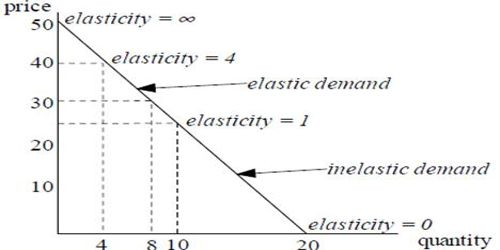# Income Elasticity and Price Elasticity of Demand measurement

Income Elasticity of Demand and Price Elasticity of Demand measurement

Income elasticity of demand: Income elasticity of demand is a measure of the relationship between a change in the quantity demanded a particular good and a change in real income. Income elasticity of demand is an economics term that refers to the sensitivity of the quantity demanded a certain product in response to a change in consumer incomes. It refers to the sensitivity of the quantity demanded a certain good to a change in real income of consumers who buy this good, keeping all other things constant. The formula for calculating income elasticity of demand is:

[Income Elasticity of demand = (% change in quantity demanded / % change in income)]

It shows how the quantity demanded changes when consumer’s income changes.

Price elasticity of demand: Price elasticity of demand is a measure of the relationship between changes in the quantity demanded of a particular good and a change in its price. It is defined as the degree of responsiveness quantity demanded of a commodity in response to change in the price of that commodity. Price elasticity of demand is a term in economics often used when discussing price sensitivity. The formula for calculating the price elasticity of demand is:

[Income Elasticity of demand = (% change in quantity demanded / % change in price)]

It shows how the quantity demanded of good changes when the price of that good changes.